Learn All Concepts of Chapter 3 Class 12 Matrices - FREE. Check - Matrices Class 12 - Full video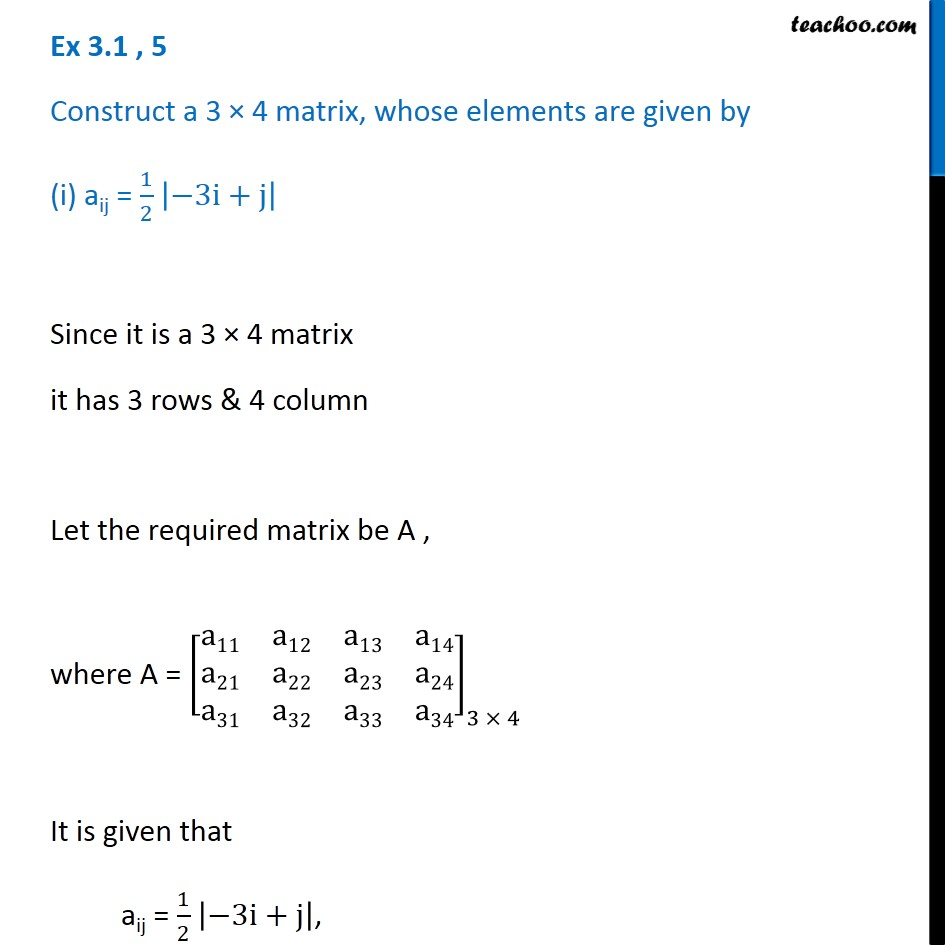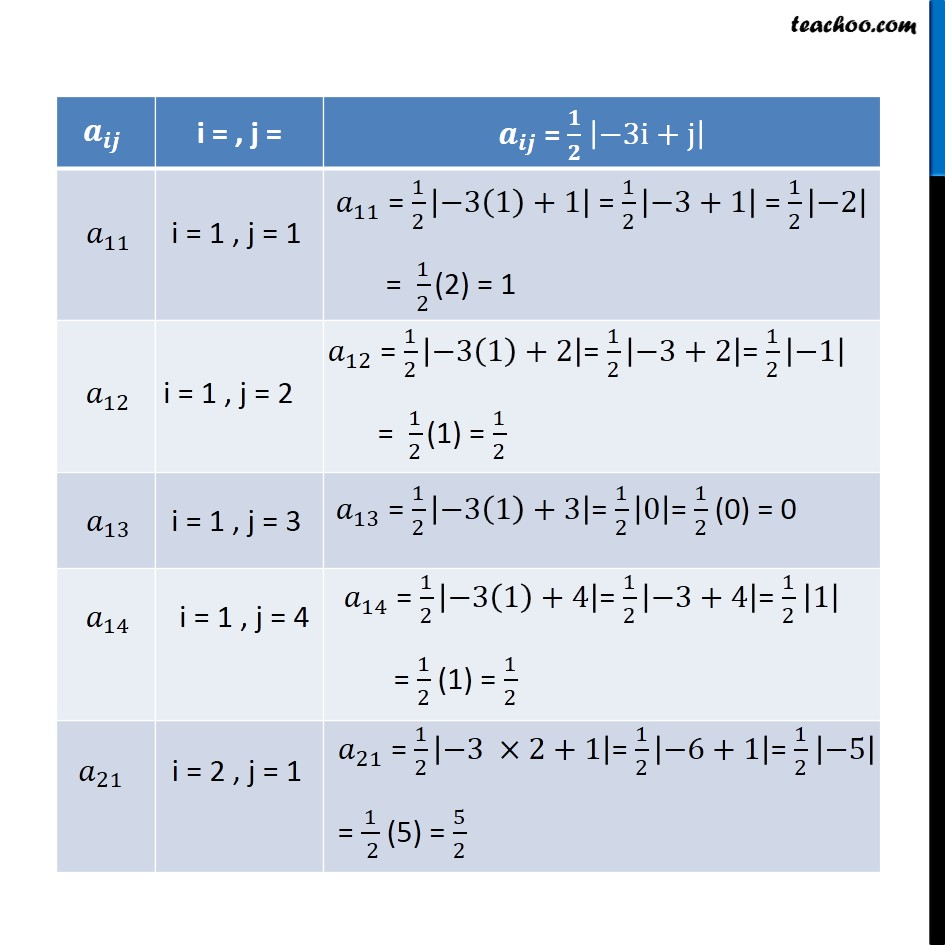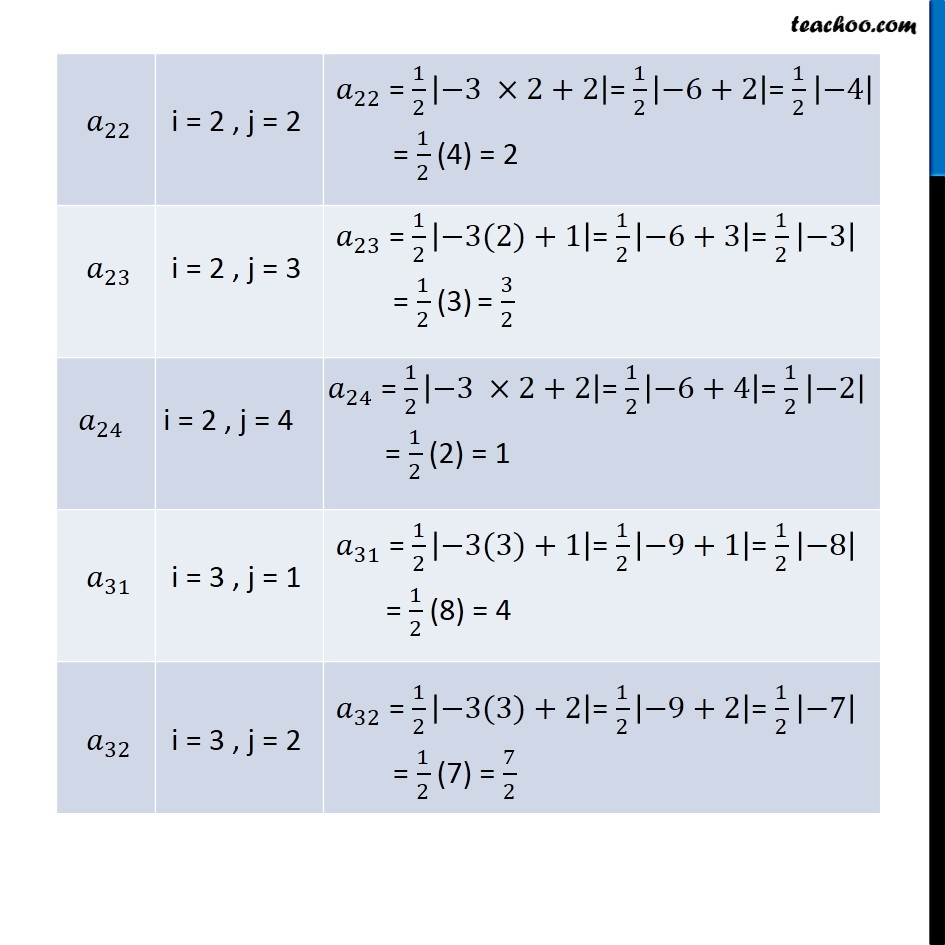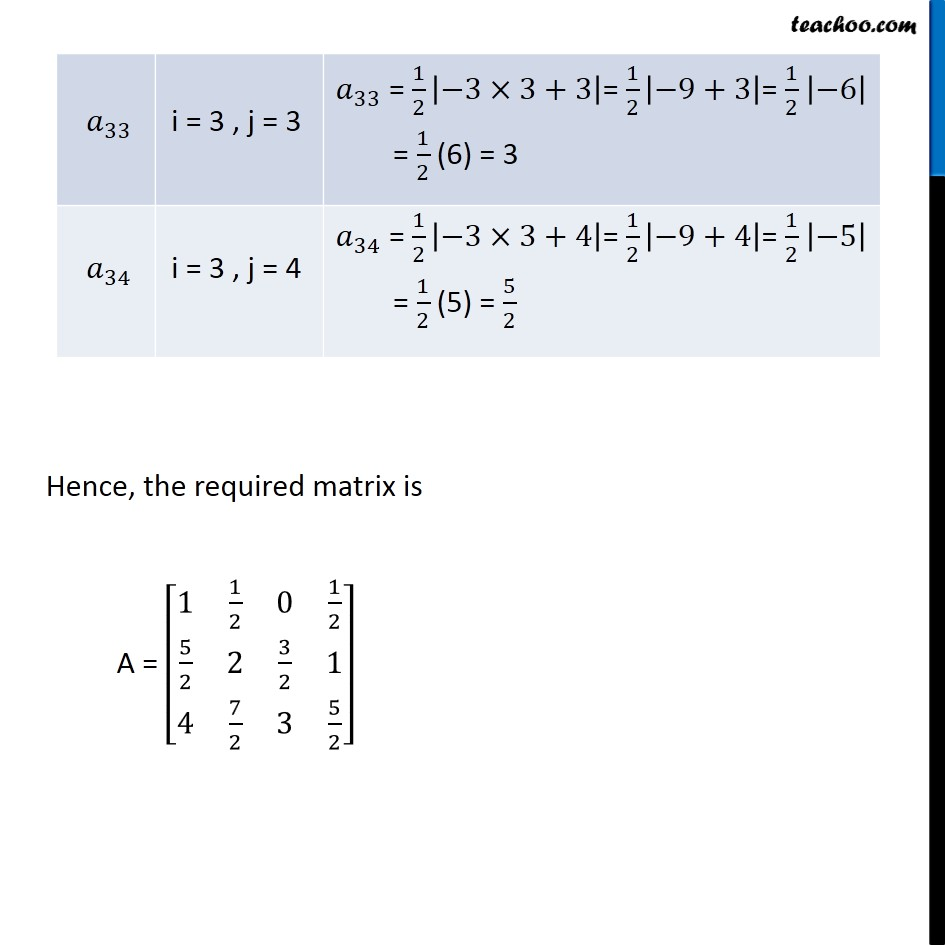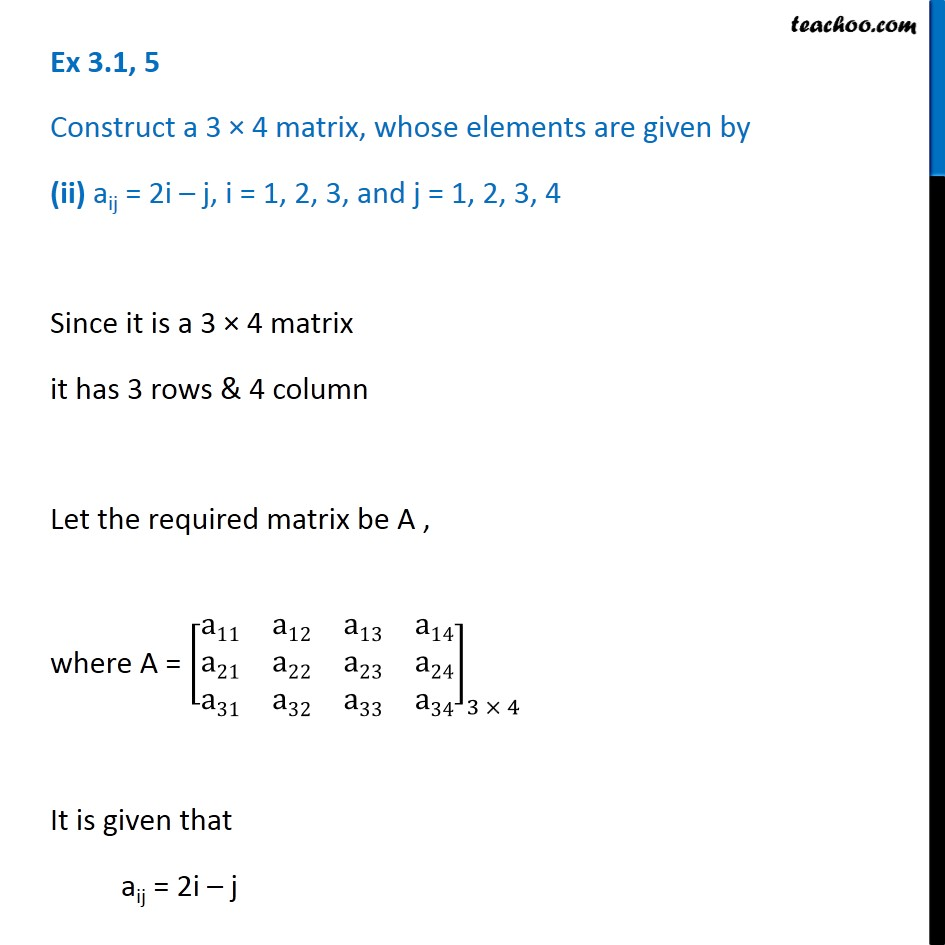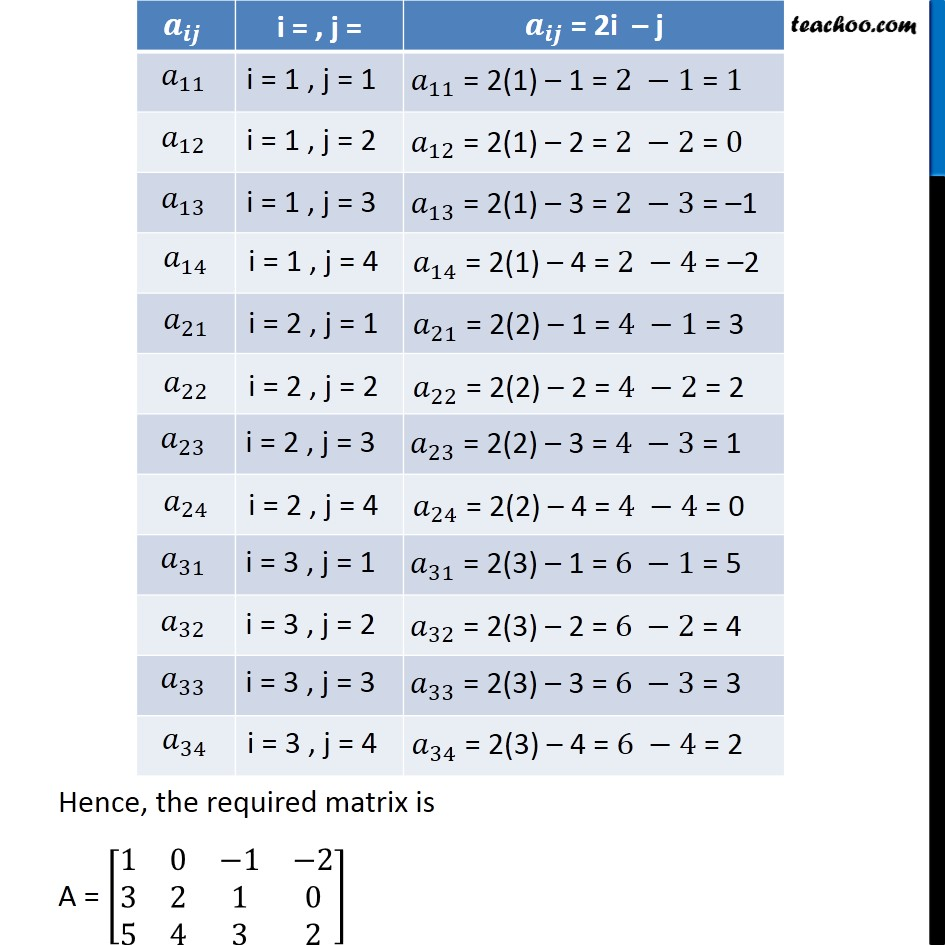1. Chapter 3 Class 12 Matrices
2. Serial order wise
3. Ex 3.1

Transcript

Ex 3.1 , 5 Construct a 3 × 4 matrix, whose elements are given by (i) aij = 1/2 |−3i+j| Since it is a 3 × 4 matrix it has 3 rows & 4 column Let the required matrix be A , where A = [■8(a11&a12&a13&a14@a21&a22&a23&a24@a31&a32&a33&a34)]_(3 × 4) It is given that aij = 1/2 |−3i+j|, Hence, the required matrix is A = [■8(1&1/2&0&1/2@5/2&2&3/2&1@4&7/2&3&5/2)] Ex 3.1, 5 Construct a 3 × 4 matrix, whose elements are given by (ii) aij = 2i – j, i = 1, 2, 3, and j = 1, 2, 3, 4 Since it is a 3 × 4 matrix it has 3 rows & 4 column Let the required matrix be A , where A = [■8(a11&a12&a13&a14@a21&a22&a23&a24@a31&a32&a33&a34)]_(3 × 4) It is given that aij = 2i – j Hence, the required matrix is A = [■8(1&0&−1&−2@3&2&1&0@5&4&3&2)]

Ex 3.1

Chapter 3 Class 12 Matrices
Serial order wise